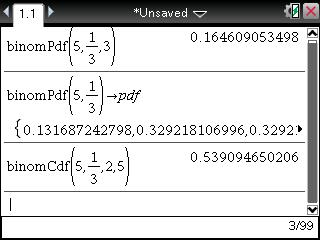# Knowledge Base

## Solution 29570: Calculating Binomial PDF and Binomial CDF on the TI-Nspire™ Family Line of Products.

### How can I calculate a Binomial PDF and Binomial CDF on the TI-Nspire family line of products?

The example below demonstrates how to calculate a binomial distribution on the TI-Nspire family line of products.

Example: A hitter has a probability of 1/3 of getting a hit each time at bat, with each at-bat independent of other at-bat. In the next times at bat:

Problem 1: What is the probability of getting exactly three hits?
Problem 2: What is the probability of getting at least two hits?

Solution 1: Computing the binomial PDF of getting exactly three hits.

• Press [home] and press [A] or choose "Add Calculator".
• Press [menu]   [D].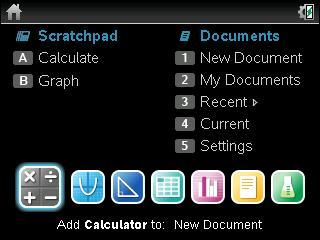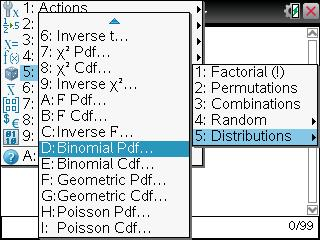• Press  [tab]  [/]  [tab]  [enter] [enter].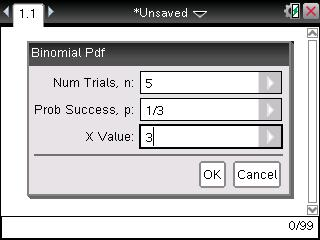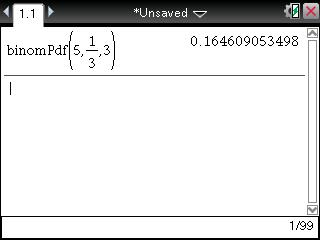NOTE: This answer means the hitter has about 16% chance of getting three hits. If X value is left blank, the entire probability distribution will be created. To view the entire list please follow steps below.

• Press [▲] [▲] [enter].
• Press [◄] [del] [del] [►].
• Press [ctrl] [sto→] [P] [D] [F] [enter].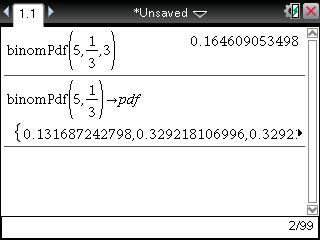• Press [▲] [=] [P] [D] [F] [enter].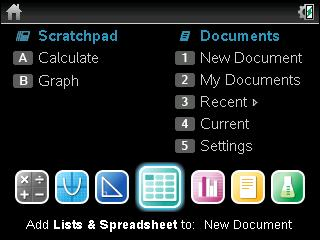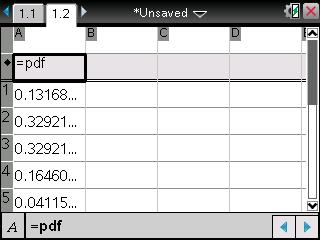Solution 2: The probability of getting at least two hits is P(2)+P(3)+P(4)+P(5).

• Press [ctrl] [◄].
• Press [menu]   [E].
• Press  [tab]  [/]  [tab]  [tab] .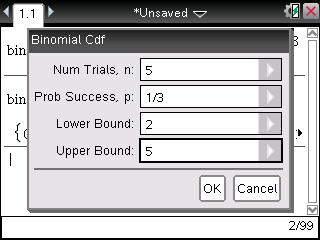• Press [enter] [enter].